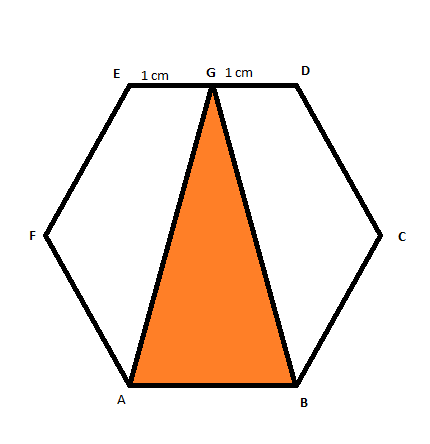# Third or Sixth Root Unity?

Geometry Level 3$ABCDEF$ is a regular hexagon with each side of $2 \text{ cm}$. Then find the area of the $\triangle$ $ABG$ to four decimal places.

×